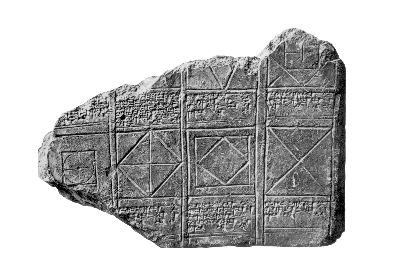## The oldest known proof

There is evidence that Pythagoras' Theorem was discovered very early by the Chinese and the Indians (refer to Heath's discussion just after I.47), but exactly how early is not known. The earliest tangible record of Pythagoras' Theorem comes from Babylonian tablets dating to around 1000 B.C. In addition to tablets containing exercises which depend on knowing at least a few specific cases, a number of tablets have been found with pictures which are in effect proofs of the Theorem in the special case where the sides of the right triangle are equal.In this case of course the Pythagoras' Theorem asserts that the ratio of the diagonal of a square to one of its sides is equal to the square root of 2.

This picture leads rather directly to the irrationality of the square root of 2. The argument we give here is variant of one from Heath's comments on Euclid (volume I, p.400).

Let s and d be the side and diagonal of the large square in the figure above. To say that s and d are commensurable, or equivalently that the ratio d/s (the square root of 2) is a rational number, means that there exists some small segment e such that d and s are both multiples of e. Let s' and d' be the side and diagonal of the smaller (red) square in the figure above. We claim that if s and d are multiples of e then so are s' and d'. But an argument about congruent triangles shows that

s' = d - s

d' = s - s'

which imply the claim.

This leads to a contradiction. The two squares are similar. Suppose the smaller one is equal to the larger one scaled by a constant c, which from the figure is clearly smaller than !. We can apply the argument over and over again to see that all lengths cn s and cn d are multiples of e. This cannot happen since cn becomes arbitrarily small.

### Reference

Otto Neugebauer, Keilschrift-Texte, Part II (especially BM 15285, Tafel 3). Springer, 1935.

Otto Neugebauer, The exact sciences in antiquity, published by Dover, 1969. Especially p. 35 and plate VI.a.